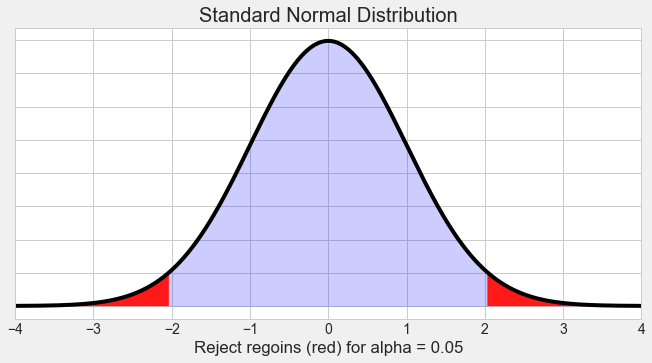1601420400

# ML: Sampling Distribution & Z-test

In the previous post, we look at how we build hypothesis testing and experiments. In this post, we start to look at the specific methods for it. The first method we are going to study is Z-test.

## Sampling Distribution

before Z-test, we need to know what is sampling distribution and how we can build it. A sampling distribution is a probability distribution of a statistic obtained from a larger number of samples drawn from a specific population. There are three ways to build this:CLT

1. Simulations, doing an experiment repeatedly. => tossing coin.
2. Analytically, we know the underlying distribution enough to build the sampling distribution. => we measure the weight or symmetry of the coin and calculate the sampling distribution with statistical techniques.
3. Central Limit Theorem, when mean is the parameter that we are going to estimate. Regardless of the underlying distribution in which the data is drawn, the sampling distribution will be a normal distribution. The mean of the sampling distribution will be the mean of the population. The standard deviation will be σ/ the root of the number of sample. Thus, larger ths sample size, narrower the sampling distribution.

Tips: The standard deviation of the sampling distribution from CLT is also called the standard error. When n is bigger than 30, we practically call it large enough because the standard error is small. However, the underlying distribution have very high variance, we need very large samples. In this case we can solve the high variance in the underlying distribution by using binning the data. It removes small variation of the data.

#z-test #machine-learning #data-science #hypothesis-testing #z-score